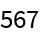#Function Repository Resource:

# HookLengths

Get the list of lists whose entries are the hook lengths of the entries of a Young diagram

Contributed by: George Beck
 ResourceFunction["HookLengths"][p] gives a list of lists of lengths p for a partition p where an entry is the hook length of the corresponding cell in the Young diagram of p.

## Details and Options

In the Young diagram of shape p, the hook for a cell c is the set of cells containing c, the cells to the right of c and the cells below c.
The hook length of a cell c is the number of cells in the hook of c.

## Examples

### Basic Examples

Here is a partition:

 In:=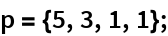This is its Ferrers diagram:

 In:=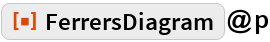Out=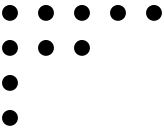These are the hook lengths for the shape p:

 In:=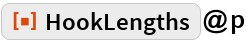Out=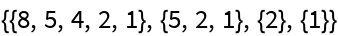This is used to format a Young diagram:

 In:=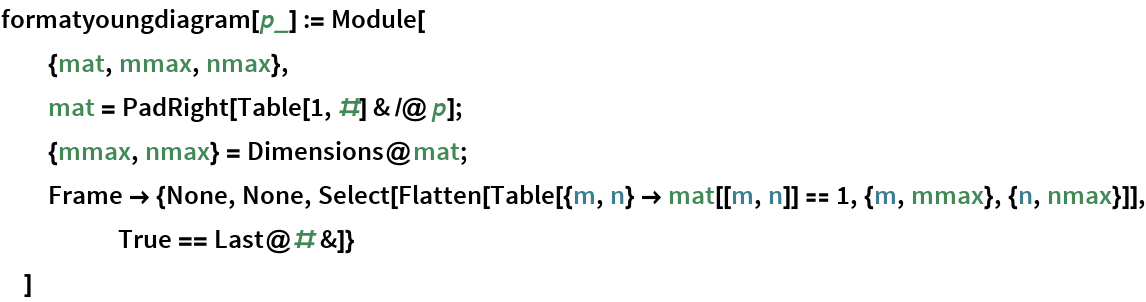The Young diagram with shape p:

 In:=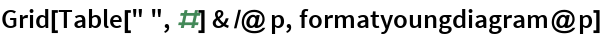Out=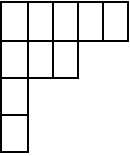A hook of length 5:

 In:=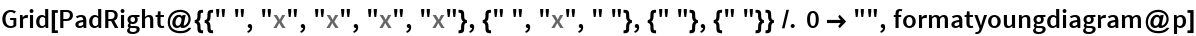Out=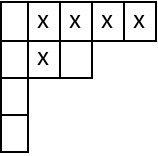The hook lengths of p:

 In:=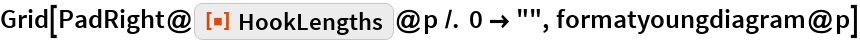Out=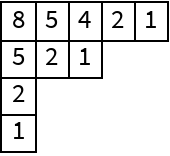### Applications

The number of standard Young tableaux for the diagram of shape p:

 In:=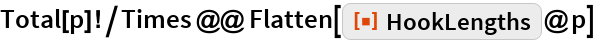Out=